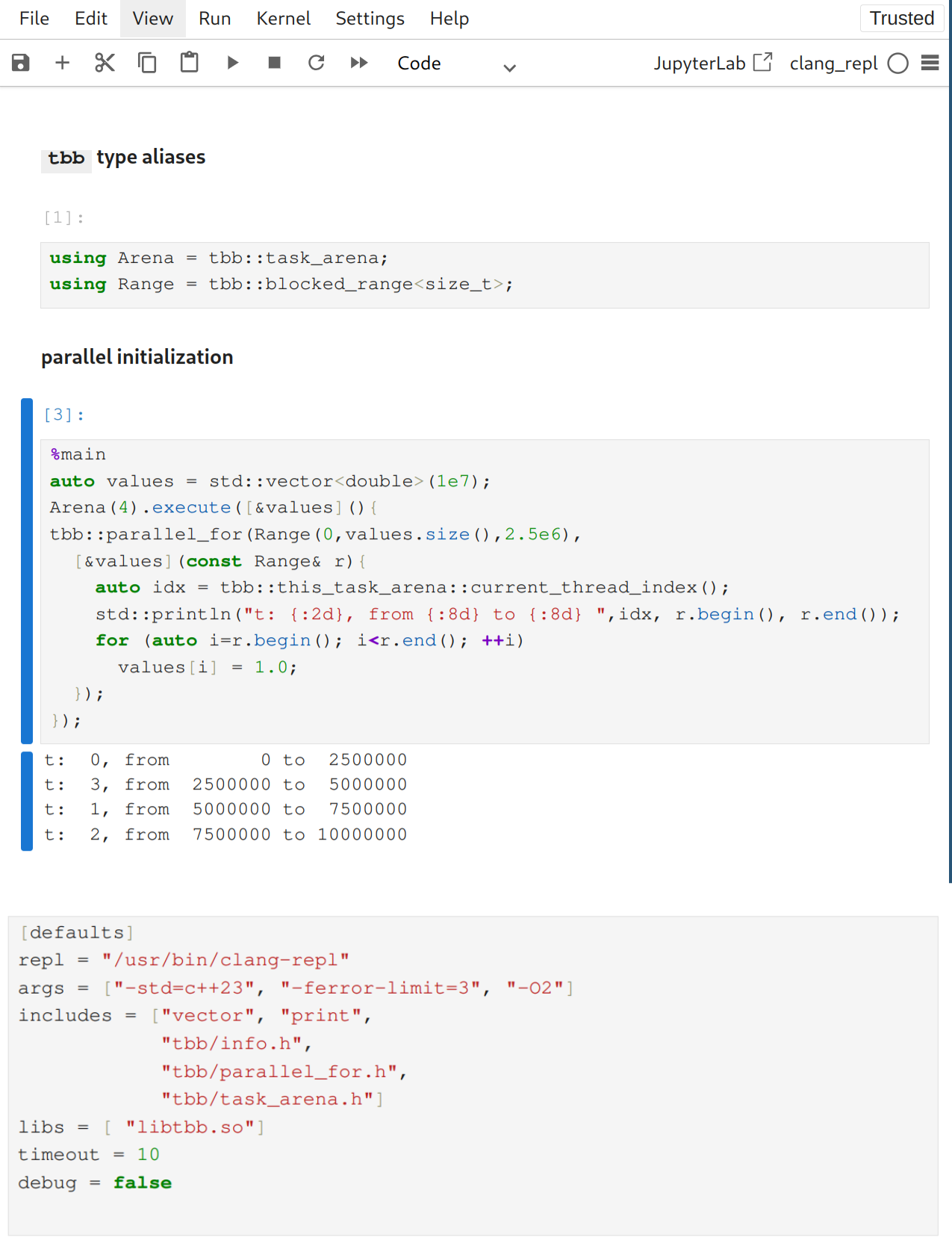Paul Manstetten Univ.Ass. Dipl.-Ing. (FH) Dr.techn. MSc Publications### Biography

Paul Manstetten was born in 1984 in Berlin, Germany. He studied Mechatronics at the University of Applied Sciences Regensburg (Dipl.-Ing.) and Computational Engineering at the University of Erlangen-Nuremberg (MSc). After three years as an application engineer for optical simulations at OSRAM Opto Semiconductors in Regensburg he joined the Institute for Microelectronics in 2015 as a project assistant. He finished his PhD studies (Dr.techn.) in 2018 and works as a postdoctoral researcher on high performance methods for semiconductor process simulation.

## Robust and Efficient Approximation of Potential Energy Surfaces using Gaussian Process Regression

The Nudged Elastic Band (NEB) method and its variants are a common choice used to identify saddle points of the potential energy surface (PES) between two given atomic configurations of a system of interest. The general idea behind the NEB method is to approximate the reaction pathway by a set of distinct atomic configurations that are connected to a string (band) by a non-physical spring force that drives iterative adjustments of the configurations to lead the band in the PES along a minimum energy pathway. In the course of this iterative saddle point search, many evaluations of the underlying physical model for the PES are required.

To evaluate the PES numerically, use of a physical model-based density functional theory (DFT) is a popular and versatile choice. Although even a single DFT approximation of the PES has high computational demands, DFT is often employed: it is an ab-initio method and so does not rely on empirical adjustments of physical parameters. However, this means that these DFT evaluations dominate the runtime of a saddle point search using the NEB method.

We explored the robustness and computational performance of using a Gaussian process regression (GPR) as a surrogate model for PES during an NEB calculation. GPR is an attractive choice as it allows one to embed analytic formulations for the prediction of derivatives - derivative information of the samples can be reflected directly - and it provides an uncertainty measure that can be used to detect invalid extrapolations. The choice of a regression kernel that is suitable for the problem is essential.

However, when integrating full derivative information in the training and prediction of the GPR model, computational demands drastically increase for larger atomic systems: the dimensions of the covariance matrix (which has to be inverted for each evaluation of the model during multidimensional hyperparameter optimization) reach a level that demands an efficient implementation of the GPR (including kernels). Ideally, the computational resources that are typically allocated for a parallelized DFT-based NEB calculation are embraced.Fig. 1: Sample regression results for observations of a Lennard-Jones potential using inverse radial distances as the similarity measure between atoms.Fig. 2: Sample regression results for observations of a Lennard-Jones potential using the common radial basis function kernel (similarity measure: distance of Euclidean atomic coordinates).Fig. 3: Dimensions for a structured covariance matrix including full partial derivative information for different system sizes.